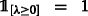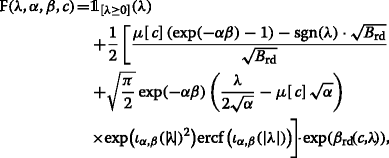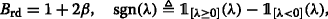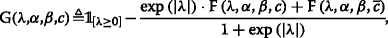# LLR-based coded performance analysis of cognitive dual-hop BICM systems

## Abstract

In this work, we analyze the performance of a cooperative two-hop cognitive relaying system using the “detect-and-forward” strategy. The cooperation between secondary users, in the underlay spectrum sharing mode, is under an interference constraint imposed by the primary network. The proposed analytical method is based on the probabilistic characterization of the bits reliability metrics given under the form of logarithmic likelihood ratios (LLRs). First, we use closed-form expressions for the probability density function of the LLRs to calculate the theoretically achievable rates and compare them with the practical throughput of a simulated turbo-coded transmission. Then, we derive tight approximations for the end-to-end coded bit error rate (BER) of the secondary transmissions, compare them with simulation results using convolutional coding schemes, and discuss their application using turbo-codes. The derivations accuracy and the system behavior are evaluated for different cooperation configurations and under different spectrum sharing conditions.

## Introduction

Cognitive relay networks (CRN), particularly with the underlay approach, have been considerably investigated as a potential way to improve secondary users throughput with optimal spectrum use. In this paper, we consider a secondary communication network consisting of a source, multiple parallel relays, and a destination, all in interaction with a primary user. We analyze the scenario where the relays communicate with the destination over independent (orthogonal) channels. Each source-relay-destination link can thus be seen as a two-hop relay channel. Relays transmit over the primary spectrum under a maximum interference temperature1 constraint.

Compared to the analysis of uncoded transmissions (based on signal-level outage probability and ergodic capacity), understanding coded systems gives more insight into the practical limits of communication schemes, with error correcting capabilities, and especially over mobile fading channels. Soft-metrics (or L values)-based analysis—which consists on calculating bits reliabilities—is one of the most common, and most efficient, approaches. In addition, the adoption of soft metrics, like the logarithmic likelihood ratios (LLR) , allows for an iterative processing at the receiver side and results in practical performance gains.

Hence, the probabilistic description of LLRs is necessary and offers a powerful analytical tool to the analysis and design of optimal end-to-end coded modulation (CM) communications and, in particular, “bit interleaved coded modulation” (BICM) schemes . Previous works in the literature adopted this LLR-based approach to derive end-to-end performance metrics such as bit error rate (BER) and throughput , and constrained capacity (both CM and BICM capacities) .

More recently, the probabilistic characterization of reliability metrics in the context of conventional relaying schemes was presented in . On the other hand, the coded performance analysis of two-hop underlay cognitive relay channels was mostly focused on the outage probability, mainly with “decode-and-forward” relaying . In , the soft-metrics-based analysis has been generalized to the more general spectrum sharing “interference-limited” cognitive context. However, the analysis remained limited to the uncoded performance. In this work, revisiting and completing the contributions in , we present and highlight a new analytical framework for the end-to-end coded performance of underlay dual-hop transmissions in terms of constrained capacity and coded error rates. We also assess the practical performance, particularly with turbo and convolutional coding schemes, in light of the presented framework.

The contributions in this work are the following. First, we apply the closed-form expressions obtained for the probability density function (PDF) of the decoding metrics in the elementary two-hop secondary network link, to derive new expressions for the BICM capacity, and a very tight upper-bound approximation of the end-to-end coded BER. Then, we present simulation results to demonstrate the accuracy of the analytical results and the performance gap between practical implementation and theoretical limits. By adressing various scenarios, the analysis provides an interesting insight into the effect of the primary user position, the relays position, and the interference limit on the end-to-end performance and, more generally, opens a new direction in the design of multihop cognitive relay systems.

The rest of this paper is organized as follows. In Section 2, we introduce the system model and assumptions. We present the PDFs of the decoding metrics in Section 3. In Section 4, we analyze the coded transmission performance as an application of the obtained results, and numerical examples illustrating the analysis are presented in Section 5. Section 6 concludes the paper.

## System model

The adopted system model of the underlay cognitive relay system is shown in Fig. 1. We assume that the primary source Sp does not cause any interference on the secondary network reception. For each single-hop link, the received signal z ij (where i{s,r}, j{r,p,d}) is given by x i as z ij=a ij·x i+n, where $$a_{\text {ij}}=h_{\text {ij}}/d_{\text {ij}}^{\nu /2}$$ denotes the channel coefficient, the amplitude of the fading coefficient |h ij| follows a Rayleigh distribution with average unit energy, d ij is the distance between i and j, n is a zero-mean additive white Gaussian noise (AWGN) with a variance N 0, and ν is the path loss exponent. In the underlay mode, secondary transmissions are allowed as long as no harmful interference impacts the primary destination Dp. This is enforced by a transmit power constraint at the secondary relay2 Rs given by

$$\mathsf{P}_{\mathrm{r}} \leq \min\left(\frac{\mathsf{I}}{\left|a_{\text{rp}}\right|^{2}},\mathsf{P}\right),$$
(1)

where I is the interference temperature and P is the maximum transmit power available at Rs. At the source, a sequence of information bits3 c are mapped onto symbols x s=μ[c]{−1,+1} from a binary phase-shift keying (BPSK) constellation and broadcasted to the relay. The average received signal-to-noise ratio (SNR) of the Ss– Rs link is thus given by $$\overline {\gamma }_{\text {sr}} = {\frac {1}{d_{\text {sr}}^{\nu }N_{0}}}$$ and the instantaneous SNR $$\gamma _{\text {sr}} = \overline {\gamma }_{\text {sr}}\left |h_{\text {sr}}\right |^{2}$$ follows an exponential distribution $$\mathrm {p}(\gamma _{\text {sr}}) = \frac {1}{\overline {\gamma }_{\text {sr}}}\exp \left (-\frac {\gamma _{\text {sr}}}{\overline {\gamma }_{\text {sr}}}\right)$$. At the secondary relay, the received signal y sr is demodulated, and the resulting binary word $$\hat {c}$$ is re-modulated creating a new signal $$x_{\mathrm {r}}=\mu _{\mathrm {r}}\left [\hat {c}\right ] \in \{-\Delta,\Delta \}$$ that is forwarded to the destination, where $$\Delta ^{2} = \min \left ({\frac {\mathsf {I}}{\left |a_{\text {rp}}\right |^{2}}},\mathsf {P}\right)$$. The instantaneous SNR is thus given by $$\gamma _{\text {rd}} = \Delta ^{2}{\frac {|a_{\text {rd}}|^{2}}{N_{0}}}$$, and its cumulative density function (CDF) can be deduced from (, Eq. (14)) applied to the special Rayleigh fading case (m 1=m 2=1). The PDF of the instantaneous SNR is thus obtained by differentiation as

$$\begin{array}{*{20}l} \mathrm{p}\!\left(\gamma_{\text{rd}}\right) = &~\beta \left(1 - \exp\left(-\alpha\beta\right)\right)\cdot\exp\left(-\beta\gamma_{\text{rd}}\right)\\ &+ \alpha \beta \exp\left(-\alpha\beta\right)\cdot\frac{\exp\left(-\beta\gamma_{\text{rd}}\right)}{\alpha + \gamma_{\text{rd}}}\\ &+ \alpha \exp\left(-\alpha\beta\right)\cdot\frac{\exp\left(-\beta\gamma_{\text{rd}}\right)}{\left(\alpha + \gamma_{\text{rd}}\right)^{2}}, \end{array}$$
(2)

where $$\alpha = {\frac {I}{N_{0}}}\cdot \left ({\frac {d_{\text {rp}}}{d_{\text {rd}}}}\right)^{\nu }$$ and $$\beta = {\frac {N_{0}}{\mathsf {P}}}\cdot d_{\text {rd}}^{\nu }$$.

At the destination, the bit-level reliability metrics can be computed under the form of LLRs as

$${}l= \log\left\{\!\frac{\text{Pr}\left(z_{\text{rd}}|\hat{c}{=}1\right)\!\left(1{-}\mathrm{P}_{\mathrm{e}}\!\left(\gamma_{\text{sr}}\right)\right) {+} \text{Pr}\left(z_{\text{rd}}|\hat{c}{=}0\right)\!\mathrm{P}_{\mathrm{e}}\! \left(\gamma_{\text{sr}}\right)}{\text{Pr}\left(z_{\text{rd}}|\hat{c}{=}0\right)\! \left(1{-}\mathrm{P}_{\mathrm{e}}\!\left(\gamma_{\text{sr}}\right)\right) {+} \text{Pr}\left(z_{\text{rd}}|\hat{c}{=}1\right)\!\mathrm{P}_{\mathrm{e}}\!\left(\gamma_{\text{sr}}\right)}\!\right\},$$
(3)

where $$\mathrm {P}_{\mathrm {e}}\!\left (\gamma _{\text {sr}}\right)=\mathrm {Q}\left (\sqrt {\gamma _{\text {sr}}}\right)$$ is the error probability at the relay and $$\mathrm {Q}(x) = {\frac {1}{\sqrt {2\pi }}\int _{x}^{\infty }\exp \left (-t^{2}/2\right)dt}$$.

## CDFs and PDFs of the reliability metrics

As derived in , the averaged CDF over γ sr is obtained as:(4)

whereif λ≥0, and 0 otherwise, $$\overline {c}$$ is the complement of c (i.e., $$\overline {c} = 1-c$$), and $$\Psi ^{-1}(x)= \left [\mathrm {Q}^{-1}\left ({\frac {1}{1 + \exp (x)}}\right)\right ]^{2}$$, Q−1(·) being the inverse Q-function, and

$$\begin{array}{*{20}l} \mathrm{U}_{\text{sr}}(\lambda)&\triangleq\int_{\Psi^{-1}(\lambda)}^{+\infty}\mathrm{Q} \left(\sqrt{\gamma_{\text{sr}}}\right)\cdot\frac{1}{\overline{\gamma}_{\text{sr}}}\cdot\exp \left(-\frac{\gamma_{\text{sr}}}{\overline{\gamma}_{\text{sr}}}\right)d\gamma_{\text{sr}}, \end{array}$$

which can be simplified for positive arguments as

$${}\mathrm{U}_{\text{sr}}(\lambda)=\!{\frac{1}{1 + \exp(\lambda)}}\cdot\exp\left(\!-\frac{\Psi^{-1}(\lambda)}{\overline{\gamma}_{\text{sr}}}\!\right)-\frac{\mathrm{Q} \left(\sqrt{B_{\text{sr}}\Psi^{-1}(\lambda)}\right)}{\sqrt{B_{\text{sr}}}},$$
(5)

where $$B_{\text {sr}}={\frac {\overline {\gamma }_{\text {sr}}+2}{\overline {\gamma }_{\text {sr}}}}$$.

The function F(λ,α,β,c) is expressed as:(6)

whereand

$$\begin{array}{*{20}l} \beta_{\text{rd}}\left(c,\lambda\right)&={\frac{\mu[c]-\text{sgn}(\lambda)\cdot\sqrt{B_{\text{rd}}}}{2}\cdot\lambda},\\ \iota_{\alpha,\beta}(\lambda)&={\frac{1}{\sqrt{2}}\cdot\left(\frac{\lambda}{2\sqrt{\alpha}} + \sqrt{B_{\text{rd}}}\cdot\sqrt{\alpha}\right)}. \end{array}$$

The expression of the average PDF (over γ sr and γ rd) of the cognitive two-hop reliability metric is obtained in  by differentiating (4) with respect to λ as:

\begin{aligned} \overline{\mathrm{p}}_{L}(\lambda|c)&=\frac{1}{\overline{\gamma}_{\text{sr}}}\!\cdot\!\mathrm{G} \left(\lambda,\alpha,\beta,c\right)\!\cdot\!\text{sgn}(\lambda)\!\cdot\!\frac{\partial \Psi^{-1}}{\partial \lambda}\!\left(\left|\lambda\right|\right)\!\cdot\!\exp\!\left(\!\!-\frac{\Psi^{-1} \left(\left|\lambda\right|\right)}{\overline{\gamma}_{\text{sr}}}\!\!\right)\\ &\quad+\frac{\exp\left(-\alpha\beta\right)}{4\sqrt{\alpha}}\cdot\mathrm{W} \!\left(\lambda,\alpha,\beta\right)\!\cdot\mathrm{V}_{\text{sr}}\left(\lambda,c\right)\cdot\exp\! \left(\!-\frac{\sqrt{\mathrm{B}_{\text{rd}}}}{2}\cdot\left|\lambda\right|\!\right)\!, \end{aligned}
(7)

where$$\begin{array}{*{20}l} {}\mathrm{V}_{\text{sr}}\left(\lambda,c\right)\triangleq&\exp\left(\frac{\mu[c]}{2}\lambda\right) \cdot\exp\left(-\frac{\Psi^{-1}\left(\left|\lambda\right|\right)}{\overline{\gamma}_{\text{sr}}}\right)\\ &-2~\! \text{sinh}\left(\frac{\mu[c]}{2}\lambda\right)\cdot\mathrm{U}_{\text{sr}}\left(\left|\lambda\right|\right)\!, \end{array}$$

and

$$\begin{array}{*{20}l} {}\mathrm{W}\left(\lambda,\alpha,\beta\right) \triangleq&-\frac{\left|\lambda\right|}{2\sqrt{\alpha}}+\sqrt{\frac{\alpha}{\mathrm{B}_{\text{rd}}}} \cdot\left(1+2\beta\exp\left(\alpha\beta\right)\right)\\ &+\sqrt{\frac{\pi}{2}}\cdot\left(\frac{\lambda^{2}}{4\alpha}-\alpha+1\right)\cdot \exp\left(\iota_{\alpha,\beta}(\left|\lambda\right|)^{2}\right)\\ &\cdot\text{ercf}\left(\iota_{\alpha,\beta}(\left|\lambda\right|)\right). \end{array}$$

Expressions (4) and (7) provide a powerful analytical tool that will simplify the analysis of the end-to-end coded performance of the analyzed system as will be shown in the next section.

## Application: analysis of coded transmissions

In this section, we propose two applications of the obtained analytical expressions. For instance, we derive analytical and numerically tractable (compared to exhaustive Monte-Carlo simulations) expressions, for both the constrained capacity and the coded BER.

### Achievable information rates

One of the most common theoretical references for coded systems is the BICM capacity  which corresponds to the normalized (by the number of channel uses) mutual information between coded bits c at the source and the reliability metrics L at the destination. In our case, it can be expressed, as in , under the form

$${}\widetilde{C} =1 + \frac{1}{2}\!\!{\underset{c\in\{0,1\}}{\sum}\!\!\int_{-\infty}^{+\infty}\!\!\overline{\mathrm{p}}_{L}\left(\lambda|c\right) \!\cdot\!\log_{2}\frac{\overline{\mathrm{p}}_{L}\left(\lambda|c\right)}{\overline{\mathrm{p}}_{L} \left(\lambda|0\right)+\overline{\mathrm{p}}_{L}\left(\lambda|1\right)}\mathrm{d}\lambda}.$$
(8)

Using the expressions obtained in (7), we are able to calculate (8) using a numerical Gaussian quadrature instead of Monte-Carlo simulations as shown in .

### Coded BER analysis

To evaluate the performance of the presented underlay scheme from a practical point of view, we use the following union bound (, Sec. 4.4) to approximate the coded BER as

$$\text{BER}\leq\text{UB}=\!\!{\overset{+\infty}{\underset{d_{\mathrm{H}}=d_{\text{free}}}{\sum}}\!\!\beta \left(d_{\mathrm{H}}\right)\cdot\text{PEP}\left(d_{\mathrm{H}}\right)},$$
(9)

where d free and β are, respectively, the minimum distance and the weight distribution spectrum of the channel code, and PEP(d H) is the pairwise error probability (PEP). Assuming that an all-ones sequence is transmitted, the PEP is given by:

$$\text{PEP}\left(d_{\mathrm{H}}\right)={\text{Pr}\left\lbrace\overset{d_{\mathrm{H}}}{\underset{j=1}{\sum}}L(j)\leq 0\right\rbrace},$$
(10)

where the random variables L(j) model the decoding metrics and j is a dummy variable. Using the saddlepoint approximation, the PEP can be approximated by (, and references therein):

$$\text{PEP}\left(d_{\mathrm{H}}\right)\approx\frac{\exp \left(d_{\mathrm{H}}\cdot\kappa(\sigma)\right)}{\sigma\sqrt{2\pi d_{\mathrm{H}}\kappa^{\prime\prime}(\sigma)}},$$
(11)

where κ(·) is the cumulant generating function (CGF) of L, σ is its “saddlepoint”, and κ ′′ (·) is the second derivative of κ(·). The CGF can thus be expressed as

$${}\kappa(x)=\log\left\{\mathbb{E}_{L}\!\left[\exp \left(x L\right)\right]\right\}=\log\int_{-\infty}^{+\infty}\!\!\overline{p}_{L}(\lambda|1)\exp \left(x\lambda\right)d\lambda,$$
(12)

and $$\overline {p}_{L}(\lambda |1)$$ is given by (7). Once again, the integral in (12) can be calculated using a Gauss-Hermite quadrature. As shown in the next section, this expression gives an accurate tool to analytically evaluate the performance of underlay coded systems.

## Numerical results

To illustrate the analysis, we assume that the relay is positioned on the line between the source and the destination and we identify its position by the ratio δ r =d sr/d sd. The position of the primary receiver Dp is identified by the ratio δ p =d rp/d rd. Considering power normalized constellations, we assume that P=1 and ν=4 for all paths.

To compare meaningfully the theoretical limits presented in  with the performance of practical coded schemes, we calculate—from a simulated transmission—the average throughput (normalized by the number of channel use) of this source-relay-destination channel given by

$${}\text{TH}(\overline{\gamma}_{\text{sd}},\delta_{r},\delta_{p}) = {\frac{1}{2}}\cdot m\cdot \rho\cdot \left[1-\text{BLER}(\overline{\gamma}_{\text{sd}},\delta_{r},\delta_{p})\right]$$
(13)

where m is the modulation order (here, m=1), ρ is the coding rate (i.e., $${\frac {1}{2}}\cdot m\cdot \rho$$ is the target spectral efficiency), and $$\text {BLER}(\overline {\gamma }_{\text {sd}},\delta _{r},\delta _{p})$$ is the block error rate at the destination when the relay is at a position given by δ r and the primary receiver is at a position given by δ p . Blocks of 4000 information bits are encoded using a turbo-code obtained by concatenating two recursive systematic convolutional encoders with generating polynomials {1,13/15}8 and a random inner interleaver. A puncturing (the parity-bit is alternatively taken out of the first and second encoder) is performed to obtain a code rate ρ=1/2 from the original rate-1/3 code. At the destination, the decoder is based on soft-input soft-output BCJR algorithm with 7 iterations.

The first analyzed scenario, depicted in Fig. 2, shows a comparison between the BICM capacity and the obtained practical throughput for different distance ratios δ p , while the relay position remains fixed at the middle point between the source and the destination δ r =0.5. It can be seen that the theoretical capacity limit increases when the distance ratio δ p is increased, with a more noticeable impact at lower ratios (δ p <1). Furthermore, and starting from a ratio δ p >2, the obtained capacity is comparable to the performance in the conventional case with no spectrum sharing constraints. As the practically achievable throughput follows the same behavior, the interesting parameter for the comparison is the SNR $$\overline {\gamma }_{\text {sd}}$$ at which the throughput attains 99% of the targeted spectral efficiency (i.e., 1/2 in the illustrated case). As an example, we can see that the analyzed secondary triplet source-relay-destination performs between 1.6 and 2.3 dB for δ p =2 and δ p =0.5, respectively, from its theoretical BICM capacity. This comparison between the BICM capacity and the throughput is presented as an illustrative example of the application of the proposed analysis approach. The gaps between theoretical and practical performance are within standard (using turbo-codes) and the optimization of the coding scheme to reduce these gaps is beyond the scope of the paper.

The second analyzed scenario, illustrated in Fig. 3, shows a comparison between the BICM capacity and the obtained practical throughput for different relay position δ r , while fixing the distance ratio identifying the primary receiver relative position at δ p =1. It is remarked that the best BICM capacity and throughput performance is reached at δ r values around 0.35, while the capacity curves with δ r =0.2 and δ r =0.5 merge together at high SNR, and the corresponding throughput performance is identical as well. This is distinctly different from the conventional case where the best performance is reached when δ r =0.5. The interference constraint makes the secondary triplet source-relay destination more dependent and more sensitive to the source-relay hop channel quality. On the other hand, as long as the system migrates to the low SNR regime; the impact of interference constraint becomes negligible against the hard radio conditions of the whole system. Consequently, the system behavior tends towards the conventional case as we can see from the BICM capacity curves.

In Fig. 4, we assess the accuracy of the obtained analytical coded BER derivations in Section 4 by comparing them with simulations for two different scenarios: (i) the ratio I/P is kept unchanged while varying the distance ratio δ (three different positions δ=0.8,1, and 2) and (ii) the distance ratio δ is kept unchanged while varying the ratio I/P (three different interference temperatures − 3, 3, and 9 dB). The encoder was implemented as a rate— 1/2 feedforward convolutional code with generating polynomials (5,7)8, so d free=5 and β(d)=2(d−5)(d−4). We adopt the value of the saddlepoint σ=1/2. From the figure, we can see that the approximation in (9) gives a tight upper-bound of the BER in the range of interest for all illustrated scenarios. Also, it is clear from the figure that the effect of the primary user’s distance ratio is more notable than the impact of the interference temperature constraint.

The upper-bound analytical approach can be applied to other coding schemes provided that the minimum distance and the weight distribution spectrum are available. With turbo-codes, although tabulated results are not as abundant as with convolutional codes, the minimum distance and the weight distribution spectrum functions can be obtained based on algorithms in the literature . In Fig. 5, we applied the same approach to a different scenario using UMTS encoder which has two recursive systematic convolutional encoders. Each convolutional encoder is an 8-state and rate 1/2, and the rate of the resulting turbo-code is 1/3. A block interleaver with length N=320 is used. The distance spectrum of UMTS turbo-codes is reported in (, Table 3) considering only the first term; so d free=24 and N free=β(d free)=1. With this limitation, we can see that the approximation in (9) gives a tight upper-bound of the BER starting from an SNR value higher than 13 dB which corresponds to a BER lower than 10−9 (which is a range of interest corresponding to typical practical/industrial performance). While the difference between simulation and analytical curves in low SNR regime is mainly due to the neglected contribution of the rest terms of the weight spectrum, the bound matches perfectly with the simulation in the high SNR regime which in fact is dominated, as well known, by the minimum distance.

Another scenario, more adapted to research purposes rather than to field application, is illustrated in Fig. 6. We used the complete weight distribution of a turbo code with blocks of 16 information bits and a 1/2 code rate (obtained via puncturing) using the encoder structure of the turbo code standardized by the Consultative Committee for Space Data Systems (CCSDS) in telemetry links . The interleaver used for permutation has been randomly generated; then, the weight spectrum was computed using a brute force matlab code (generating all the possible coded sequences from the 216 input block possibilities, and calculating the Hamming distances with a reference all-zero sequence). The obtained results show that the calculated BER is a lower-bound compared to the simulated curve, with a progressive match at higher SNR values. Here, the decoding algorithm is sub-optimal because of the reduced code size; with standard code sizes, simulations would follow and match, earlier and better, the analytical curve. This is different from the scenario in Fig. 5, where the gap is mainly coming from the approximations in the calculated BER.

As an extension of this work, more illustrative examples can be developed with standard longer block lengths using special algorithms for generating the complete weight spectrum.

## Conclusion

In this paper, a soft-metrics-based analysis of BICM cognitive underlay dual-hop systems was presented. The statistics of the LLRs were expressed in closed-form when detect-and-forward is the adopted relaying strategy. Achievable information rates and coded BER expressions—numerically computable and more tractable than heavy Monte-Carlo simulations—were also presented as applications of the obtained analytical statistical tools. For different scenarios, the accuracy of the derivations was confirmed by simulation results and the gap between practical performance and theoretical rates was assessed. The proposed analysis method provides a powerful tool for the design and optimization of coded cooperative systems. It can also be adopted in the more general context of multihop communications, very present in the future device-to-device and machine-type 5G communications.

## Endnotes

1 In the underlay mode, interference temperature (expressed in dBm) refers to the maximum received interference signal strength tolerated by the primary destination to authorize secondary transmissions.

2 We suppose that the secondary source transmission does not impact the primary node reception; only the secondary relay transmits under the cognitive interference constraint. In practice, this assumption can be adopted when the secondary source Ss is located far away from the primary destination Dp; when there is an obstruction between Ss and Dp; when Ss uses a beamforming transmission away from Dp; or when Ss uses a different frequency band than Rs.

3 For the sake of brevity, although we consider a BICM transmission, the analysis will consider the information bits directly even though it is the interleaved version of the bits that is in direct contact with the channel. However, since the interleaver is assumed to be deterministic and known from both sides, making abstraction of the interleaving does not impact the generality of the analysis.

## References

1. AJ Viterbi, JK Omura, Principles of Digital Communications and Coding (Mack Graw Hill, New York, 1979).

2. G Caire, G Taricco, E Biglieri, Bit-interleaved coded modulation. IEEE Trans. Inf. Theory. 44(3), 927–946 (1998).

3. L Szczecinski, F-K Diop, M Benjillali, A Ceron, R Feick, in Proc. IEEE Global Commun. Conf. (GLOBECOM). BICM in HARQ with mapping rearrangement: capacity and performance of practical schemes (IEEEWashington, D.C, 2007), pp. 1410–1415.

4. M Benjillali, L Szczecinski, Detect-and-forward in two-hop relay channels: a metrics-based analysis. IEEE Trans. Commun. 58(6), 1729–1736 (2010).

5. J Lee, H Wang, JG Andrews, D Hong, Outage probability of cognitive relay networks with interference constraints. IEEE Trans. Wireless Commun. 10(2), 390–395 (2011).

6. C Zhong, T Ratnarajah, K-K Wong, Outage analysis of decode-and-forward cognitive dual-hop systems with the interference constraint in Nakagami-m fading channels. IEEE Trans. Veh. Technol. 60(6), 2875–2879 (2011).

7. Z El-Moutaouakkil, K Tourki, KA Qaraqe, S Saoudi, in Proc. IEEE Vehicular Technology Conference (VTC-Fall). Exact outage probability analysis for relay-aided underlay cognitive communications (IEEEQuébec City, 2012), pp. 1–5.

8. L Luo, P Zhang, G Zhang, J Qin, Outage performance for cognitive relay networks with underlay spectrum sharing. IEEE Commun. Lett. 15(7), 710–712 (2011).

9. A Fedoul, M Benjillali, in Proc. International Workshop on Codes, Cryptography, and Communication Systems (IWCCCS’14). Cognitive detect-and-forward relay channels: soft metrics and performance analysis (IEEEEl Jadida, 2014), pp. 113–117.

10. L Szczecinski, A Alvarado, Bit Interleaved Coded Modulation: Fundamentals, Analysis and Design (Wiley, Hoboken, 2015).

11. MAU Bhuiyan, MS Hosain, S Rahman, in Proc. 5th WSEAS International Conference on Applied Electromagnetics, Wireless and Optical Communications. Distance spectrum analysis of third generation turbo codes (WSEAS, 2005), pp. 69–74.

12. CCSDS, TM Synchronization and Channel Coding. Blue Book, CCSDS 131.0-B-2 (2011).

### Funding

The authors declare that there has been no special funding given to this work.

## Author information

Authors

### Contributions

AF (first author) made substantial contributions to the conception and design, acquisition of data, and analysis and interpretation of data and has been involved in drafting the manuscript. MB (second author) made substantial contributions to the conception and design and analysis and interpretation of data and has been involved in revising the manuscript critically for important intellectual content. Both authors read and approved the final manuscript.

### Corresponding author

Correspondence to Anas Fedoul.

## Ethics declarations

### Competing interests

The authors declare that they have no competing interests.

### Publisher’s Note

Springer Nature remains neutral with regard to jurisdictional claims in published maps and institutional affiliations.

## Rights and permissions